## Saturday, June 23, 2018

### "Oh! This is Cross-Multiplication....!!"

I had given them enough time to struggle and figure out how to evaluate 'x' from such equations, where variable is in the denominator: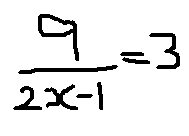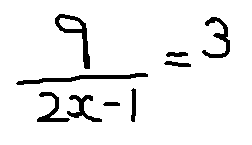But when I found that they were unable to do this for long, I decided to intervene. In the above problem, it's easy to visualize that 2x-1 has to be 3, so that we get quotient as 3, and for this 2x should be 4 and hence x=2.

But I was aware that this game would not be always so easy. For ex., what if it is -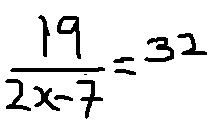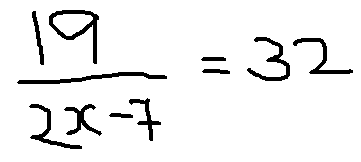It's not so easy to solve this problem using the approach above (Why?)

So the objective was to accomplish a general strategy. Meanwhile, I was also aware that they are very comfortable with equations having variable in the numerator.

So this is how it went:

How do you solve:  x/4 = 2

They immediately said, x = 8

Okay, but how?

"We have expressed division in the form of multiplication..."

Correct. And how else would you arrive at the 2nd equation from the 1st one -- using any rule of equality?

"Yes, we multiplied both the sides by 4.."

And why do you need to do so?

"Because we want to keep the 'x' alone...and its now divided by 4.... so only when we multiply it by 4, its division effect will get nullified."

Fine.... If you understand this form, then lets consider this now:
(only the 1st equation of the image below was written)

"We will multiply by x on both the sides.."

Why?

"To remove the denominator from the equation..."

And they solve it further as above. (divided the equation by 3 to get the value of 1x...)

Fine... Lets consider this equation now....
(only the 1st equation of the image below was written)

Immediately, they said -- "Multiply by 'x+1' on both the sides...."

So tell me what should I write....   They dictated, & I simply wrote...

Now?

"Multiply  3 and x+1 "

I am waiting for you to tell me.....

" 7 = 3x + 3 "

I paused again....... they understood.....

" 4 = 3x "

Pause...

" x = 4/3 "

( I didn't push them for the reason this time...)

Fine... let's take one more case now.....

And I just kept writing what they dictated to me.....

Finally, I drew an arrow (as you see above) and asked them to notice something....

" Oh.... It's a Cross Multiplication...."

I looked at their faces, one by one, while some were either still glued to this magical appearance or wondering about its How part  :-)

So... what happened...?

"Sir, that's how its taught in the school...."

Then why weren't you using it to solve the equation...?

"We forgot...."

And what could be the reason for this?

They laughed.... "Sir, we didn't know the reason for this rule...we had not understood this..."

So now?

"Now we understood this... We won't forget..."  this came smilingly with some shades of embarrassment...  :)

(But was it 'they' who need to be embarrassed for this?)

All this was written on our floor and they began noting this down in their note-books....

Meanwhile, I made up these problems for them on the board for practice:

Do you feel I have made these 3 problems randomly or there is some thought process invested in formulating them? (to answer this, you might like to have a re-look at all of the problems discussed above)

I gave them some time to solve..... And after few minutes, this happened....

Student-1

Student-2

They were surprised in the beginning when they arrived at the equation, 34x = 0. I think this was the first time, they were solving an equation where the value of the variable turns out to be 0. If you notice, they have also verified it by substituting the value of x in the original equation.

Student-3

Yes, as you see they solved it well, even simplified it.However my objective to give them this problem was something else....I just drew the arrow again... and waited for their response...

One of them shouted -- "Sir, its getting inverted..."

Other said -- "Inverse on both the sides.."

Without any discussion at this point, I simply, gave them this problem: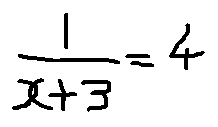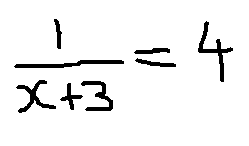So how would have students done this problem? And what would have been the line of discussion further?   We will see that in Part-2 of this post :-)

Meanwhile, I would like to know  ---

a) Your views about the trajectory followed by the teacher to make the students learn to solve Linear equations of :   a / f(x) = b / g(x)    -- What would have You done if your student/ child would have expressed his/ her inability to solve such problems?

b)  Your experiences about the so called 'Cross multiplication' method?  - and how it was arrived here...

c) Was it a right decision/ practice of me to expose them to this Inverse taking method of simplification now? Why so?

Waiting to hear from you,

Thanks and Regards
Rupesh Gesota

"I touch the future..... I teach."

## Monday, June 11, 2018

### When students are not directly fed the text-book methods - A.P. - Part-2

Hello folks,

So as I had said, I am back again with the Part-2 of this story :-)

Hope you remember about the onset of an unusual activity in our class? - my (lower grade) students have started doing (& enjoying) Maths from (higher-grade) text-books. Its an unusual activity not just because of the different in the class-levels, but because we had never used any text-books till now!  :-))

In the previous post, I had shared about their exploration of problems based on AP (arithmetic progression) and I am  happy that many of you liked their different approaches to solve one of the problems. For those, who haven't read the previous post yet, this is the link:

So after solving some more AP-based problems (should we really call them problems? :-), we  moved on to GP (Geometric progression).

In mathematics, a geometric progression, also known as a geometric sequence, is a sequence of numbers where each term after the first is found by multiplying the previous one by a fixed, non-zero number called the common ratio. For example, the sequence 2, 6, 18, 54, ... is a geometric progression with common ratio 3.

As we did in AP, here too I asked them to figure out the formula for -
a) nth term of GP and then,
b) sum of first n terms of GP

They found the former quickly but needed some help in the second case.

Then I gave them around 4-5 problems at a stretch, out of which first few were quite easy.

This one took some time, but I am glad they could solve it.

I would again suggest you to (try to) solve this on your own first....
....
....
....
....
....
....
....

Following are the solutions of students:

Method-1: by Vaishnavi

Method-2: by Jitu

Method-3 - by Rohit

Yes, Rohit's method is somewhat similar to that of Vaishnavi's. But the reason I have shared it because he has worked out on his own and secondly, his representation is little different than that of Vaishnavi.

1) How did you solve this problem?

Thanks and Regards
Rupesh Gesota

PS: These students are from grade-7 and 8 Marathi medium government school and are part of a maths enrichment program- MENTOR. To know more, check www.supportmentor.weebly.com

## Saturday, June 9, 2018

### When students are not directly fed the text-book methods - A.P. - Part-1

I have been Playing Maths with a bunch of marathi-medium municipal school students after their school-hours.

As I can now see them approach and solve quite challenging (out of the text-book) problems comfortably, I decided, for a change, to now pick up their text-book for a while and see what unfolds...Of course, I cannot make them solve the problems from the school text-books of their age (they have surpassed it long back). So I  directly picked up their SSC (grade-10) Algebra text-book. (They have just cleared their grade-7 and grade-8 exams) The first chapter was about Sequence (AP, GP). I was aware that this would be a cakewalk for them. So I just rushed through the terminologies and asked them to derive the formula for Nth term of Arithmetic Progression (AP)

(For those who are unaware or have forgotten what AP is --- let me give you couple of example, instead of its definition :)
i) 2, 5, 8, 11, 14, ......
ii) 3, -7, -17, -27, ....
iii) 5, 10, 12, 17, 19, ....
iv) 2, 20, 200, 2000, .....

Only (i) and (ii) are APs while (iii) and (iv) are not APs.. (common difference (d) = 3 in the 1st case and d = -10 in the 2nd case)

So what I asked them was to make a formula for nth term of AP i.e. in a given AP, if you wish to 'quickly' find the 200th term, 5369th term, etc without listing down all the middle terms, then one can do this using its formula.

They didn't take more than half a minute derive the required result. It was correct.
Tn = T1 + (n-1)d)

So now I immediately asked them to derive the formula for Sum of first nth terms of AP (Sn)

The book was in my hand and hence I saw its method. I was wondering if this method will click to them or will they do something else, but I was very sure they will crack it. And in almost a minute all hands were up.

No wonder, they had used a different method.... But the main thing to wonder was they all had used the same method :-)

I forgot to take the snap of their work. So I am typing down what they did:

Sn =  T1  + T2 + T3 +T4 + ...
=  T1  + (T1 + d) + (T1 + 2d) + (T1 + 3d) +....
=  nT1 + d + 2d + 3d + ....
=  nT1 + d (1 + 2 + 3 +....)
=  nT1 + d (n-1) n / 2
=  n [ T1 + d(n-1)/2 ]

If you observe they have used the formula for sum of first few (n-1) natural numbers for deriving an expression for Sn.

However the text book derivation does not use this method and formula (in fact the formula for sum of first n natural no.s is derived later in the book using the formula for Sn).. And that's also fine.

So I first appreciated them for their accuracy but then challenged them to get the expression for Sn without using the formula for sum of first few natural numbers. I asked them - what if you didn't know the formula that you have used in this derivation?

To this, one of them replied  - "Sir, we would have derived it the way we had done it long back.."

"Hmm... And how had you done that?"

"By pairing up.... First and last numbers.... second and second-last... and so on...."

"Yes, so why don't use the same idea here too?"

They looked at me puzzled...

So after a minute, I just showed them the method given in their text book.

They studied it and they responded like -- "Sir, isn't this the same pairing method?''  :-)

Then, I gave them some text-book problems just for practice (were they 'problems'? :-) Luckily, the last one among them was a little better.

problem: 4 terms are in AP. Product of 1st and 4th terms is 45. Sum of 2nd and 3rd terms is 18. Find the terms.

I would suggest you to (try to) solve this problem before reading further...

....
....
....
....
....

Yes -- because the text-book was again in my hand, I saw the method. However, let me also confess that I would have used the same method because this is what was directly taught to me till drill and kill.

Thankfully, I recovered from being an instructor and don't do this damage anymore :)
And you know what?  This not only saves my effort but also helps me learn interesting methods from them :-))

Method-1: By Jitu

He argued that since 3 x d x t1 and 45 are multiples of 3, hence (t1)^2 should also be multiple of 3. Hence it can take only two values: 9 and 36 to get the required sum 45. Substituting (t1)^2 as 36 does not satisfy the equation and substituting as 9 does satisfy. He solved further to arrive at the required AP.

He was the first to complete the problem. To engage him, I gave him another similar problem, but of 3 consecutive numbers. (sum of 3 no.s. is 27 & their product is 504) and he was quick to solve this one too:

Method-2 - By Sahil (original 4 numbers problem)

He too argued similarly about the addition equation. Since '2a' and '3d' sum up to an Even number, and '2a' is already Even, so '3d' too should be Even. Hence 'd' should be Even. (did you understand this? :-)

So then he found the possible values for d. He also said that 'd' cannot be 0 since that would make all terms equal, which is in turn not possible 45 (given as product of two terms) is not a perfect square !  He also said that 'd' cannot be '6' because that would make a=0 leading to the product of 1st and 4th terms as 0, thus contradicting the given information.

He then found the corresponding 2 values for a, substituted each pair in the equation.
(a,d) = (6,2) didn't satisfy but (3,4) did satisfy. He then got the required sequence.

Method-3 - by Kanchan

She first told me that Sum of 2nd & 3th terms would be same as the Sum of 1st and 4th terms.

When I asked her why, she said - its obvious. :)  1st term is less than 2nd term by the same amount as the 4th term is more than 3rd term.

Did you get this?   :-) :-)

I was happy she could visualize this relation, however I challenged her to prove it while she was writing on the board, and if you notice, she has done it partially.

What drove me crazy was her next move.She went into Quadratic Equation.
"we know the sum of two numbers, we know the product of same two numbers.. So we can make an equation, and find its roots.."

Hope you will take some time to study her solution.

(let me mention that quadratic equation is formally taught in 10th, that too in a dry way... she has not only used quadratics while in 8th but has also applied that knowledge in another topic altogether!)

And her method, gave us both the possible sequences - 3,7,11,15   and  15,11,7,3 which was not the case with other methods.

Finally, I asked them if they wanted to know how its solved in the text-book. And they all were curious to know :-)

So I simply copy pasted this method on the board, leaving for them to analyze and understand.... And they could easily do so....

I waited for them to study this approach. And then -

"So if I give you another problem -- but this time of 3 consecutive numbers in AP, then how will you solve it?"

"We will then take those terms as  a-d, a, a+d"

"Oh ! you will use this text-book method?"

"Yes sir, this is an interesting and even an efficient one!"

I could see them smiling.

"So I should have directly showed this method to you, isn't it?"

"No.... then how would we think & discover our own methods?", Vaishnavi reacted. :-)

..... (Part-2 soon)
Thanks & Regards
Rupesh Gesota
www.supportmentor.weebly.com